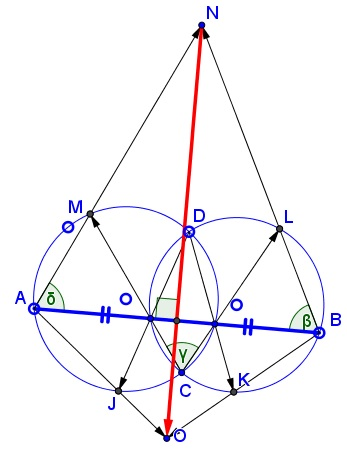# A Straightedge Construction of the Midpoint of a Chord Common to Two Circles

### Problem### Solution

In the diagram below, $\angle \beta=\angle \gamma,$ as two inscribed angles subtended by the same chord $OL$ (not shown.) For the same reason, $\angle \gamma=\angle\delta,$ so that, by transitivity, $\angle \beta=\angle\delta.$It follows that $AN=BN.$ Similarly, $AO=BO.$ It follows that $AOBN$ is a kite in which the diagonal $NO$ is perpendicular to the diagonal $AB$ and passes through the midpoint of the latter.

### Acknowledgment

The problem is by Thanos Kalogerakis who kindly informed me of the problem and later communicated to me the solution by Nikoz Fragkakis.